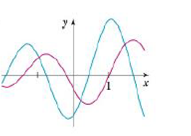Chapter 2.8, Problem 48E

Chapter
Section
Textbook Problem

The graphs of a function f and its derivative f' are shown. Which is bigger, f'( –1) or f"(l)?To determine

To Identify: The bigger quantity among f(1) and f(1) with proper explanation.

Explanation

Note:

The derivative is zero where the function has a horizontal tangent.

Graph:

The given graph of f and f is shown in Figure 1.

Observation:

From Figure 1 it is observed that the point where curve c2 has functional value 0 is the same point where graph of curve c1 has horizontal tangent.

By note, c2 is the derivative of the graph curve c1.

Thus, c1=f and c2=f.

Calculation:

From Figure 1, f(1)>0 as the graph of f lies above the x-axis for x=1.

Thus, f(1)>0 (1)

From Figure 1, f(x) has horizontal tangent at x=1.

By note, the value of the derivative of f at x=1 is 0

Still sussing out bartleby?

Check out a sample textbook solution.

See a sample solution

The Solution to Your Study Problems

Bartleby provides explanations to thousands of textbook problems written by our experts, many with advanced degrees!

Get Started

In Exercises 7-12, refer to the following figure. 12. Which point has a y-coordinate that is equal to zero?

Applied Calculus for the Managerial, Life, and Social Sciences: A Brief Approach

Evaluate the integral. xtan2xdx

Calculus (MindTap Course List)

Given: m9=2x+17m11=5x94 Find: x

Elementary Geometry For College Students, 7e

Evaluate: 12x21xdx. a) 322 b) 2+21 c) 4+2 d) 14

Study Guide for Stewart's Single Variable Calculus: Early Transcendentals, 8th

Evaluate each expression. (84)

College Algebra (MindTap Course List)

Explain how inter-rater reliability is established.

Research Methods for the Behavioral Sciences (MindTap Course List)